Courses
Courses for Kids
Free study material
Free LIVE classes
More

# Find the Missing DigitsLIVE
Join Vedantu’s FREE Mastercalss

## Overview of Missing Digits

Do you know what missing digits actually are? Missing digits are the digits that are missed within the given series of numbers with similar variations among them. You have to find the number that is missing. The simple method of finding these missing digits is by identifying the similar changes in the numbers and filling the digits in the given series.

Now you must be curious about how to find the missing digits. But after reading this article and solving some practice questions, you will be able to comprehend the topic of missing digits in addition, subtraction, and multiplication.

## Find the Missing Digits in Addition

To find the missing digits in addition, follow the steps in the given example: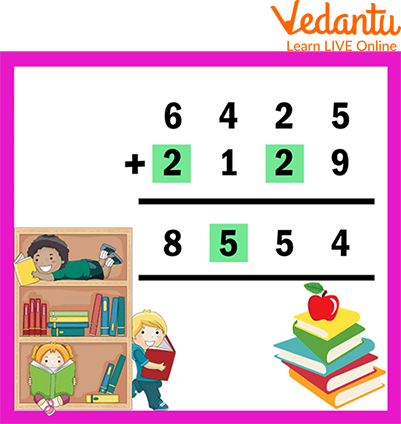• Step 1: Add the ONES: 5 + 9 = 14

• Step 2: Regroup as 1 Tens and 4 Ones

• Step 3: Add the TENS: 2 + 1 carry over = 3

• Step 4: Write 2 in the box to make 3 + 2 = 5

• Step 5: Add the HUNDREDS: 4 + 1 = 5

• Step 6: Add the THOUSANDS: 6 + 2 = 8

• Step 7: Write 2 in the box. This is how the missing number will be filled.

## Find the Missing Digits in Subtraction

To find the missing digits in subtraction, follow the steps in the given example: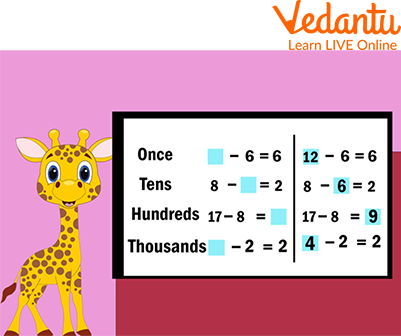Missing Digits in Subtraction

Here we can see that we have one missing digit from which 6 have been subtracted to get the result as 6. So, if we subtract 6 from the 12, we get 6. Hence, the missing digit for once is 12. i.e. 12 - 6 = 6.

For tens, here we can see that we have one missing digit. We have 8, and if we subtract 8 from a missing digit, we will get the result as 2. So, if we subtract 6 from 8, we will get the result as 2. i.e. 8 - 6 = 2

For hundreds, here we can see that we have one missing digit. We have 17 and 8, and if we subtract 17 from 8, we will get the result as 9. So, the missing digit will become 9. i.e. 17 - 8 = 9

For thousands, here we can see that we have one missing digit from which 2 have been subtracted to get the result as 2. So, if we subtract 2 from the 4, the result we get is 2. Hence, the missing digit for once is 4. i.e. 4 - 2 = 2.

## Find the Missing Number in Multiplication

To find the missing digits in multiplication, follow the steps in the given example: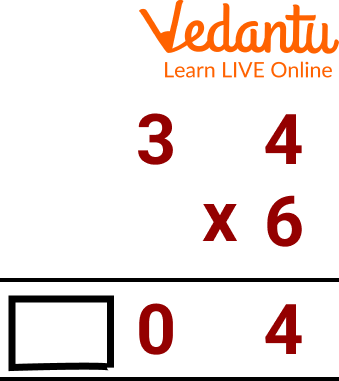Example for Multiplication

• Step 1: First of all check where the missing place is, here we can see that we have the missing place in the result.

• Step 2: Multiply $4 \times 6$ and we get 24. So, 4 will be at ones place, and now we have 2 in carry.

• Step 3: Now, we will multiply, $6 \times 3$ and we get 18. As we have 2 carry also, so we will add 2 in it. It will become 20.

• Step 4: Hence, the missing digit is 2.

## Notice the Pattern and Find the Missing Digits

Select 2 or 3 terms in the text in which a rule will be applied to find the missing number. For example: If you have five numbers in a series then pick the first three numbers to check if any rule is to be applied.

While choosing the number to check the rule, select the number which is easy to operate. These include terms like factors of 2, 3, 5, or 10. Check the series with some common rules such as the sum of the terms, squares, cube, or other.

To find the missing digits in a pattern, follow the steps in the given example:

## Find the Missing Numbers 1, 2, 6, 24

The given sequence has 4 terms. We will check which rule is applied by picking the first 3 terms. The second number in the sequence is 2 and the first number is 1, which means 1 is either added or 2 is multiplied to obtain the second term.

The third term is 6 which we get from 2 by multiplying it with 3. So, now we have 1 x 1, 1 x 2, 2 x 3, and 6 x 4. Thus, we have identified the pattern and accordingly, the last term will be 24 x 5= 120.

Hence, the missing number is 120.

## Solved Examples

Q1. Find the missing digit marked with a yellow box.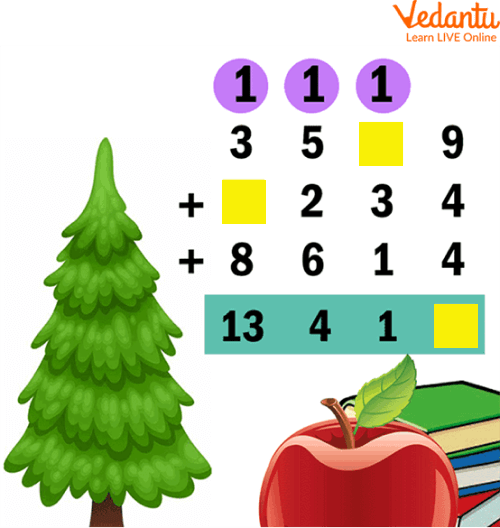Ans:

Steps:

I: Add the one place that is 9 + 4 + 4 = 17. Put 7 in the box and carry 1 to the tens.

II: Add tens place leaving the box so that 1 + 3 + 1 = 5. If 5 + 6 = 11, then we get 1 in tens place. put 6 in the box and carry 1 to the hundreds.

III: Add the hundreds place including the carried 1 so that 1 + 5 + 2 + 6 = 14. Now we get 4 in the hundreds place and now, carry 1 to the thousands.

IV: Add thousands leaving the box so that 1 + 3 + 8 = 12. 12 + 1 = 13. Put 1 in the box.Q2. Find the missing digit marked with a yellow box.Ans:

Steps:

I: Add ones leaving the box. 4 + 6 = 10 and 10 + 5 = 15, therefore we get 5 in the ones place. Write 5 in the box and carry 1 to the tens place.

II: Add tens place. 1 + 3 + 4 + 9 = 17. Write 7 in the tens place and carry 1 to the hundreds place.

III: Add the hundreds place leaving the box. 1 + 5 +3 = 9. 9 + 2 = 11, then we get 1 in the hundreds place. Write 2 in the box. Carry 1 to the thousands place.

IV: Add thousands place leaving the box. 1 + 6 + 1 = 8; 8 + 1 = 9. Write 1 in the box.## Practice Questions

Find the Missing Digits in Addition:

I. Fill □ by the correct digit.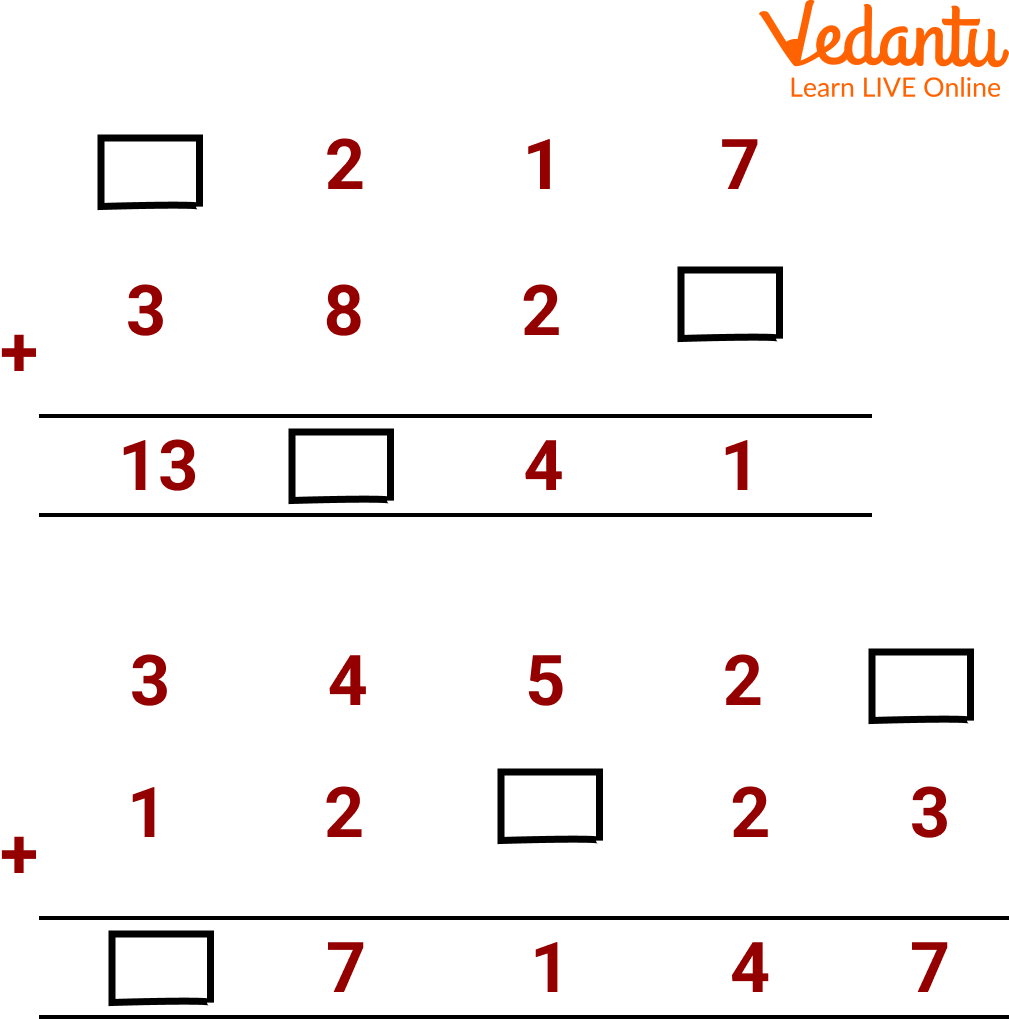Fill in the Box

Ans: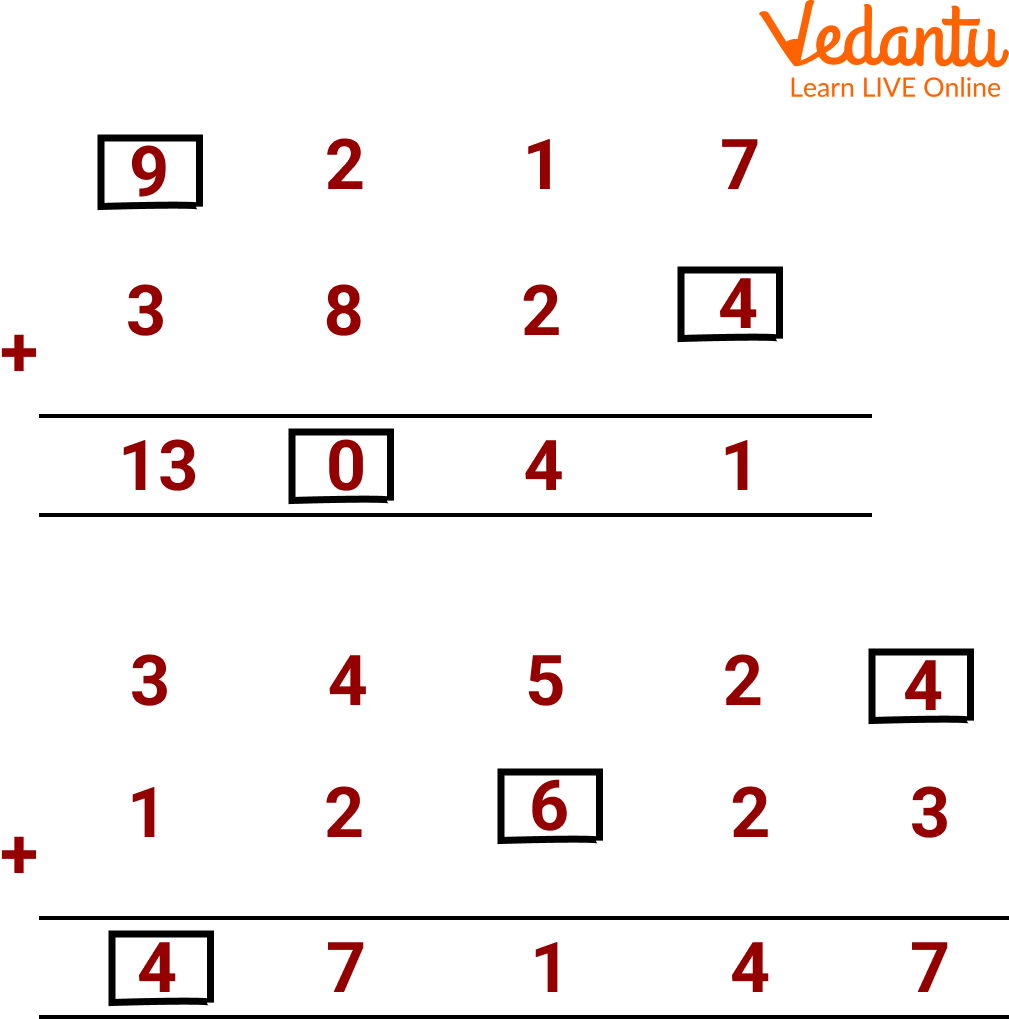Fill in the Box

Find the Missing Digits in Subtraction

II. Fill □ by the correct digit.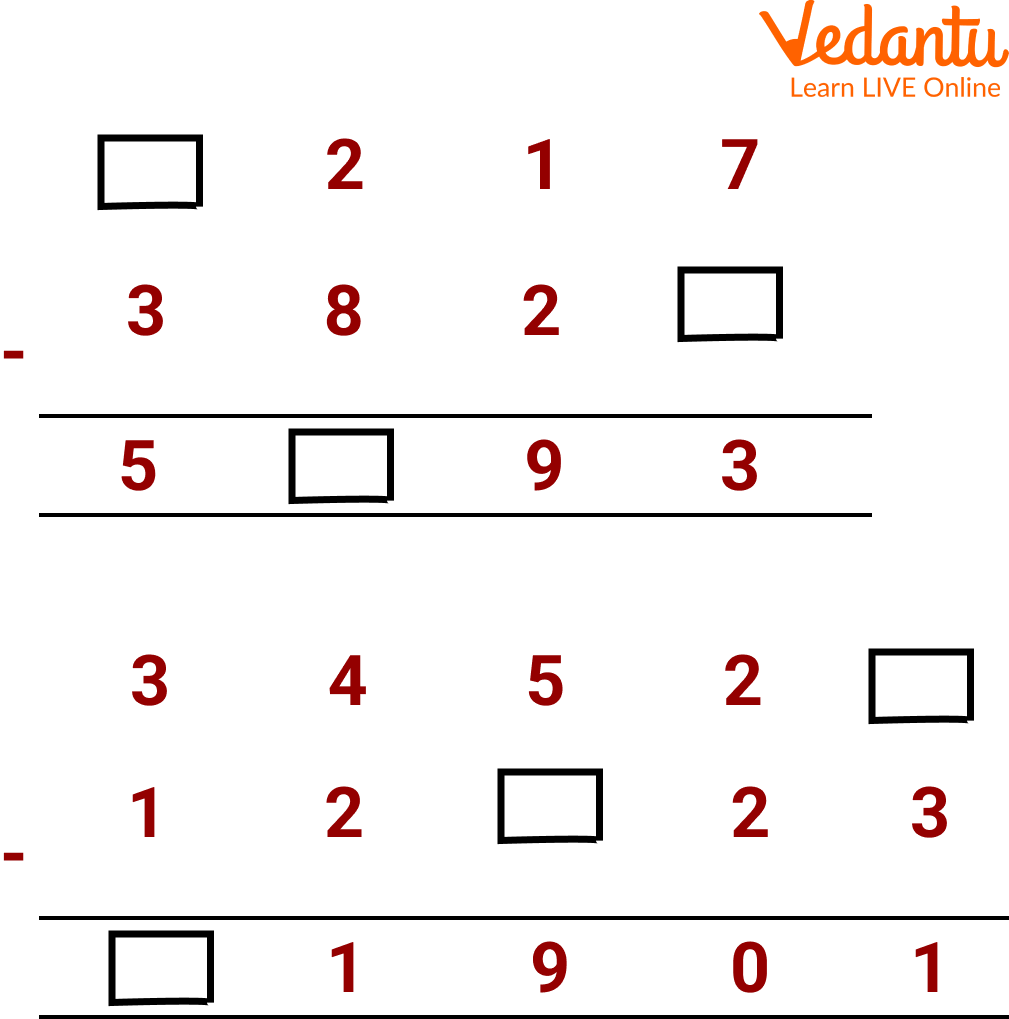Fill in the Box

Ans: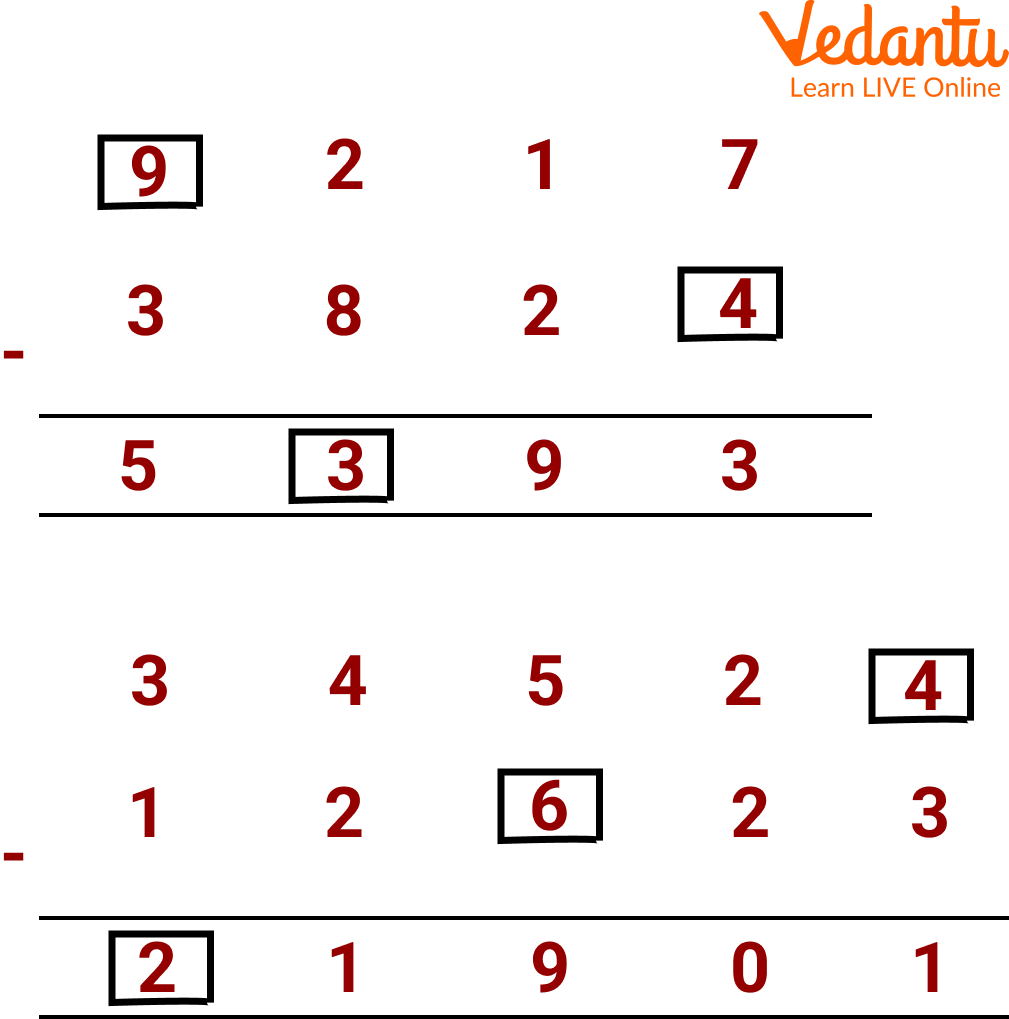Fill in the Box

Find the Missing Digits in Multiplication: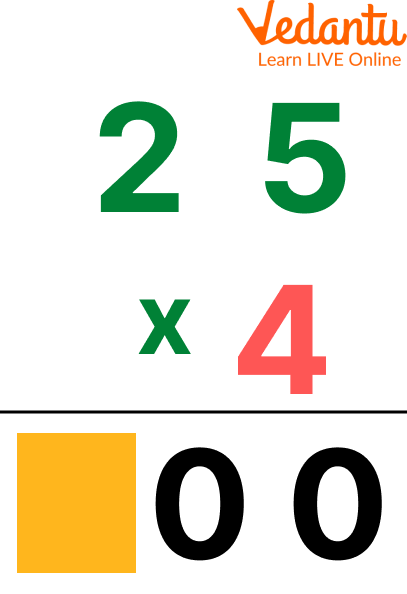Fill in the Box

Ans: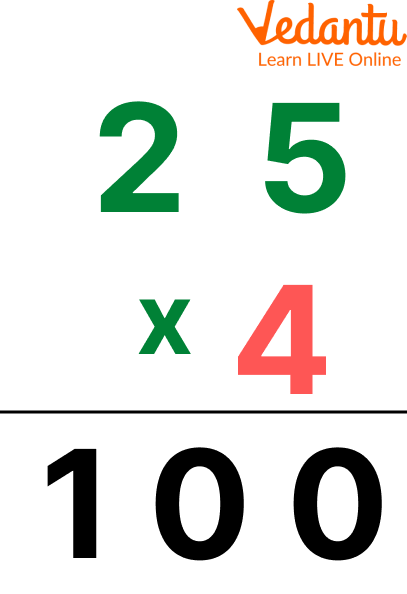Fill in the box

## Summary

Missing numbers are the numbers that have been missed in a given series of a number or digits with similar differences among them. The method of filling in these missing numbers is stated as finding similar changes or rules between those numbers and filling the missing terms in the specific series and places. You can also find the missing digits by identifying the pattern of the sequence. Keep practising and solving the missing digits worksheets and you will be able to solve such questions easily.

Last updated date: 24th Sep 2023
Total views: 90.3k
Views today: 1.90k

## FAQs on Find the Missing Digits

1. What are the benefits of missing numbers games?

The task of identifying missing numbers games helps kids build mental skills while also adding to their fine motor practice.

2. How do you solve a puzzle problem?

The following are the basic steps to solve logical puzzles:

Take a look at the question. Get an idea about the theme of the problem. Select the data that is giving you some idea out of the total information given. Also, select the data which helps you in ruling out different possibilities.

3. How to look for missing numbers in a table?

Observing the pattern between the numbers in the table can help you find the missing numbers in a table.